$$\newcommand{\id}{\mathrm{id}}$$ $$\newcommand{\Span}{\mathrm{span}}$$ $$\newcommand{\kernel}{\mathrm{null}\,}$$ $$\newcommand{\range}{\mathrm{range}\,}$$ $$\newcommand{\RealPart}{\mathrm{Re}}$$ $$\newcommand{\ImaginaryPart}{\mathrm{Im}}$$ $$\newcommand{\Argument}{\mathrm{Arg}}$$ $$\newcommand{\norm}{\| #1 \|}$$ $$\newcommand{\inner}{\langle #1, #2 \rangle}$$ $$\newcommand{\Span}{\mathrm{span}}$$

# 8.R: Further Applications of Trigonometry (Review)

•• Contributed by Jay Abramson
• Principal Lecturer (School of Mathematical and Statistical Sciences) at Arizona State University
• Publisher: OpenStax CNX
$$\newcommand{\vecs}{\overset { \rightharpoonup} {\mathbf{#1}} }$$ $$\newcommand{\vecd}{\overset{-\!-\!\rightharpoonup}{\vphantom{a}\smash {#1}}}$$$$\newcommand{\id}{\mathrm{id}}$$ $$\newcommand{\Span}{\mathrm{span}}$$ $$\newcommand{\kernel}{\mathrm{null}\,}$$ $$\newcommand{\range}{\mathrm{range}\,}$$ $$\newcommand{\RealPart}{\mathrm{Re}}$$ $$\newcommand{\ImaginaryPart}{\mathrm{Im}}$$ $$\newcommand{\Argument}{\mathrm{Arg}}$$ $$\newcommand{\norm}{\| #1 \|}$$ $$\newcommand{\inner}{\langle #1, #2 \rangle}$$ $$\newcommand{\Span}{\mathrm{span}}$$ $$\newcommand{\id}{\mathrm{id}}$$ $$\newcommand{\Span}{\mathrm{span}}$$ $$\newcommand{\kernel}{\mathrm{null}\,}$$ $$\newcommand{\range}{\mathrm{range}\,}$$ $$\newcommand{\RealPart}{\mathrm{Re}}$$ $$\newcommand{\ImaginaryPart}{\mathrm{Im}}$$ $$\newcommand{\Argument}{\mathrm{Arg}}$$ $$\newcommand{\norm}{\| #1 \|}$$ $$\newcommand{\inner}{\langle #1, #2 \rangle}$$ $$\newcommand{\Span}{\mathrm{span}}$$

## 8.1: Non-right Triangles: Law of Sines

For the exercises 1-5 assume $$\alpha$$ is opposite side $$a$$, $$\beta$$ is opposite side $$b$$, and $$\gamma$$ is opposite side $$c$$. Solve each triangle, if possible. Round each answer to the nearest tenth.

1) $$\beta =50^{\circ}, a=105, b=45$$

Not possible

2) $$\alpha =43.1^{\circ}, a=184.2, b=242.8$$

3) Solve the triangle.$$C=120^{\circ}, a=23.1, c=34.1$$

4) Find the area of the triangle.Figure below. Find the distance of the plane from point $$A$$ and the elevation of the plane.distance of the plane from point $$A:2.2$$ km, elevation of the plane: $$1.6$$ km

## 8.2: Non-right Triangles - Law of Cosines

1) Solve the triangle, rounding to the nearest tenth, assuming $$\alpha$$ is opposite side $$a$$, $$\beta$$ is opposite side $$b$$, and $$\gamma$$ s opposite side $$c: a=4, b=6,c=8$$$.$

2) Solve the triangle in the Figure below, rounding to the nearest tenth.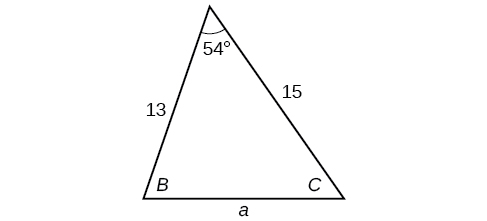$$B=71.0^{\circ},C=55.0^{\circ},a=12.8$$

3) Find the area of a triangle with sides of length $$8.3$$, $$6.6$$, and $$9.1$$.

4) To find the distance between two cities, a satellite calculates the distances and angle shown in the Figure below (not to scale). Find the distance between the cities. Round answers to the nearest tenth.$$40.6$$ km

## 8.3: Polar Coordinates

1) Plot the point with polar coordinates $$\left ( 3,\dfrac{\pi }{6} \right )$$.

2) Plot the point with polar coordinates $$\left ( 5,\dfrac{-2\pi }{3} \right )$$.3) Convert $$\left ( 6,\dfrac{-3\pi }{4} \right )$$ to rectangular coordinates.

4) Convert $$\left ( -2,\dfrac{3\pi }{2} \right )$$ to rectangular coordinates.

$$(0,2)$$

5) Convert $$(7,-2)$$ to polar coordinates.

6) Convert $$(-9,-4)$$ to polar coordinates.

$$(9.8489,203.96^{\circ})$$

For the exercises 7-9, convert the given Cartesian equation to a polar equation.

7) $$x=-2$$

8) $$x^2+y^2=64$$

$$r=8$$

9) $$x^2+y^2=-2y$$

For the exercises 10-11, convert the given polar equation to a Cartesian equation.

10) $$r=7\cos \theta$$

$$x^2+y^2=7x$$

11) $$r=\dfrac{-2}{4\cos \theta +\sin \theta }$$

For the exercises 12-13, convert to rectangular form and graph.

12) $$\theta =\dfrac{3\pi }{4}$$

$$y=-x$$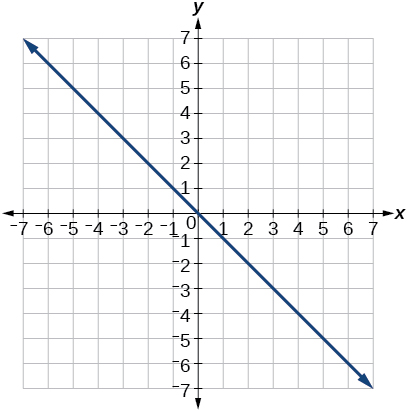13) $$r=5\sec \theta$$

## 8.4: Polar Coordinates - Graphs

For the exercises 1-5, test each equation for symmetry.

1) $$r=4+4\sin \theta$$

symmetric with respect to the line $$\theta =\dfrac{\pi }{2}$$

2) $$r=7$$

3) Sketch a graph of the polar equation $$r=1-5\sin \theta$$. Label the axis intercepts.4) Sketch a graph of the polar equation $$r=5\sin (7\theta )$$.

5) Sketch a graph of the polar equation $$r=3-3\cos \theta$$## 8.5: Polar Form of Complex Numbers

For the exercises 1-2, find the absolute value of each complex number.

1) $$-2+6i$$

2) $$4-3i$$

$$5$$

Write the complex number in polar form.

3) $$5+9i$$

4) $$\dfrac{1}{2}-\dfrac{\sqrt{3}}{2}i$$

$$\mathrm{cis}\left (-\dfrac{\pi }{3} \right )$$

For the exercises 5-6, convert the complex number from polar to rectangular form.

5) $$z=5\mathrm{cis}\left (\dfrac{5\pi }{6} \right )$$

6) $$z=3\mathrm{cis}(40^{\circ})$$

$$2.3+1.9i$$

For the exercises 7-8, find the product $$z_1 z_2$$ in polar form.

7) \begin{align*} z_1 &= 2\mathrm{cis}(89^{\circ})\\ z_2 &= 5\mathrm{cis}(23^{\circ}) \end{align*}

8) \begin{align*} z_1 &= 10\mathrm{cis}\left ( \dfrac{\pi }{6} \right )\\ z_2 &= 6\mathrm{cis}\left ( \dfrac{\pi }{3} \right ) \end{align*}

$$60\mathrm{cis}\left ( \dfrac{\pi }{2} \right )$$

For the exercises 9-10, find the quotient $$\dfrac{z_1}{z_2}$$ in polar form.

9) \begin{align*} z_1 &= 12\mathrm{cis}(55^{\circ})\\ z_2 &= 3\mathrm{cis}(18^{\circ}) \end{align*}

10) \begin{align*} z_1 &= 27\mathrm{cis}\left ( \dfrac{5\pi }{3} \right )\\ z_2 &= 9\mathrm{cis}\left ( \dfrac{\pi }{3} \right ) \end{align*}

$$3\mathrm{cis}\left ( \dfrac{4\pi }{3} \right )$$

For the exercises 11-12, find the powers of each complex number in polar form.

11) Find $$z^4$$ when $$z=2\mathrm{cis}(70^{\circ})$$

12) Find $$z^2$$ when $$z=5\mathrm{cis}\left ( \dfrac{3\pi }{4} \right )$$

$$25\mathrm{cis}\left ( \dfrac{3\pi }{2} \right )$$

For the exercises 13-14, evaluate each root.

13) Evaluate the cube root of $$z$$ when

14) Evaluate the square root of $$z$$ when $$z=25\mathrm{cis}\left ( \dfrac{3\pi }{2} \right )$$.

$$5\mathrm{cis}\left ( \dfrac{3\pi }{4} \right )$$, $$5\mathrm{cis}\left ( \dfrac{7\pi }{4} \right )$$

For the exercises 15-16, plot the complex number in the complex plane.

15) $$6-2i$$

16) $$-1+3i$$## 8.6: Parametric Equations

For the exercises 1-4, eliminate the parameter $$t$$ to rewrite the parametric equation as a Cartesian equation.

1) $$\begin{cases} & x(t)= 3t-1\\ & y(t)= \sqrt{t} \end{cases}$$

2) $$\begin{cases} & x(t)= -\cos t\\ & y(t)= 2\sin ^2t \end{cases}$$

$$x^2+\dfrac{1}{2}y=1$$

3) Parameterize (write a parametric equation for) each Cartesian equation by using $$x(t)=a\cos t$$ and $$y(t)=b\sin t$$ for $$\dfrac{x^2}{25}+\dfrac{y^2}{16}=1$$.

4) Parameterize the line from $$(-2,3)$$ to $$(4,7)$$ so that the line is at $$(-2,3)$$ at $$t=0$$ and $$(4,7)$$ at $$t=1$$.

$$\begin{cases} & x(t)= -2+6t\\ & y(t)= 3+4t \end{cases}$$

## 8.7: Parametric Equations - Graphs

For the exercises 1-, make a table of values for each set of parametric equations, graph the equations, and include an orientation; then write the Cartesian equation.

1) $$\begin{cases} & x(t)= 3t^2\\ & y(t)= 2t-1 \end{cases}$$

2) $$\begin{cases} & x(t)= e^t\\ & y(t)= -2e^{5t} \end{cases}$$

$$y=-2x^5$$3) $$\begin{cases} & x(t)= 3\cos t\\ & y(t)= 2\sin t \end{cases}$$

4) A ball is launched with an initial velocity of $$80$$ feet per second at an angle of $$40^{\circ}$$ to the horizontal. The ball is released at a height of $$4$$ feet above the ground.

1. Where is the ball after $$3$$ seconds?
2. How long is the ball in the air?
1. $$\begin{cases} & x(t)= (80\cos (40^{\circ}))t\\ & y(t)= -16t^2+(80\sin (40^{\circ}))t+4 \end{cases}$$
2. The ball is 14 feet high and 184 feet from where it was launched.
3. $$3.3$$ seconds

## 8.8: Vectors

For the exercises 1-2, determine whether the two vectors, $$u$$ and $$v$$, are equal, where $$u$$ has an initial point $$P_1$$ and a terminal point $$P_2$$, and $$v$$ has an initial point $$P_3$$ and a terminal point $$P_4$$.

1) $$P_1=(-1,4), P_2=(3,1), P_3=(5,5), P_4=(9,2)$$

2) $$P_1=(6,11), P_2=(-2,8), P_3=(0,-1), P_4=(-8,2)$$

not equal

For the exercises 3-4, use the vectors $$u=2i-j,v=4i-3j$$, and $$w=-2i+5j$$ to evaluate the expression.

3) $$u-v$$

4) $$2v-u+w$$

$$4i$$

For the exercises 5-6, find a unit vector in the same direction as the given vector.

5) $$a=8i-6j$$

6) $$b=-3i-j$$

$$-\dfrac{3\sqrt{10}}{10}i-\dfrac{\sqrt{10}}{10}j$$

For the exercises 7-11, calculate $$u\cdot v$$.

7) $$u=-2i+j$$ and $$v=3i+7j$$

8) $$u=i+4j$$ and $$v=4i+3j$$

$$16$$

9) Given $$v=\left \langle -3,4 \right \rangle$$ draw $$v$$, $$2v$$, and $$\dfrac{1}{2}v$$.

10) Given the vectors shown in the Figure below, sketch $$u + v$$, $$u − v$$ and $$3v$$.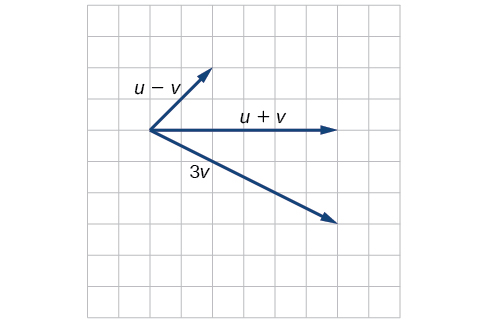11) Given initial point $$P_1=(3,2)$$ and terminal point $$P_2=(-5,-1)$$$,$write the vector $$v$$ in terms of $$i$$ and $$j$$. Draw the points and the vector on the graph.

## Practice Test

1) Assume $$\alpha$$ is opposite side $$a$$, $$\beta$$ is opposite side $$b$$, and $$\gamma$$ is opposite side $$c$$. Solve the triangle, if possible, and round each answer to the nearest tenth, given $$\beta =68^{\circ},b=21,c=16$$.

$$\alpha =67.1^{\circ}, \gamma =44.9^{\circ}, a=20.9$$

2) Find the area of the triangle in the Figure below. Round each answer to the nearest tenth.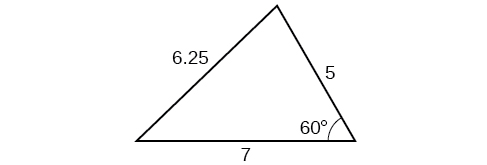3) A pilot flies in a straight path for $$2$$ hours. He then makes a course correction, heading $$15^{\circ}$$ to the right of his original course, and flies $$1$$ hour in the new direction. If he maintains a constant speed of $$575$$ miles per hour, how far is he from his starting position?

$$1712$$ miles

4) Convert $$(2,2)$$ to polar coordinates, and then plot the point.

5) Convert $$\left ( 2,\dfrac{\pi }{3} \right )$$ to rectangular coordinates.

$$(1,\sqrt{3})$$

6) Convert the polar equation to a Cartesian equation: $$x^2+y^2=5y$$.

7) Convert to rectangular form and graph:$$Answer \(y=-3$$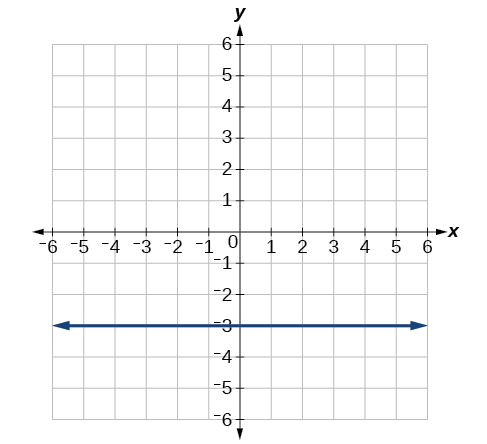8) Test the equation for symmetry: $$r=-4\sin(2\theta )$$.

9) Graph $$r=3+3\cos \theta$$.10) Graph $$r=3-5\sin \theta$$.

11) Find the absolute value of the complex number $$5-9i$$.

$$\sqrt{106}$$

12) Write the complex number in polar form: $$4+i$$.

13) Convert the complex number from polar to rectangular form: $$z=5\mathrm{cis}\left ( \dfrac{2\pi }{3} \right )$$

$$\dfrac{-5}{2}+i\dfrac{5\sqrt{3}}{2}$$

Given $$z_1=8\mathrm{cis}(36^{\circ})$$ and $$z_2=2\mathrm{cis}(15^{\circ})$$$,$evaluate each expression.

14) $$z_1 z_2$$

15) $$\dfrac{z_1}{z_2}$$

$$4\mathrm{cis}(21^{\circ})$$

16) $$(z_2)^3$$

17) $$\sqrt{z_1}$$

$$2\sqrt{2}\mathrm{cis}(18^{\circ}), 2\sqrt{2}\mathrm{cis}(198^{\circ})$$

18) Plot the complex number $$-5-i$$ in the complex plane.

19) Eliminate the parameter $$t$$ to rewrite the following parametric equations as a Cartesian equation: $$\begin{cases} & x(t)= t+1\\ & y(t)= 2t^2 \end{cases}$$

$$y=2(x-1)^2$$

20) Parameterize (write a parametric equation for) the following Cartesian equation by using $$x(t)=a\cos t$$ and $$y(t)=b\sin t : \dfrac{x^2}{36}+\dfrac{y^2}{100}=1$$

21) Graph the set of parametric equations and find the Cartesian equation: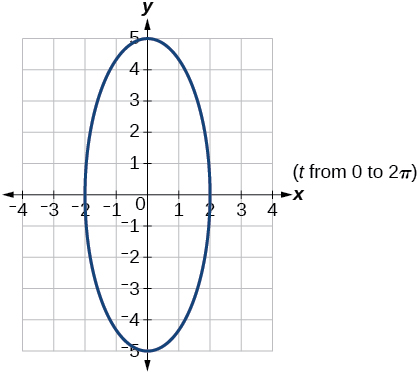22) A ball is launched with an initial velocity of $$95$$ feet per second at an angle of $$52^{\circ}$$ to the horizontal. The ball is released at a height of $$3.5$$ feet above the ground.

1. Where is the ball after $$2$$ seconds?
2. How long is the ball in the air?

For the exercises 23-26, use the vectors $$u = i − 3j$$ and $$v = 2i + 3j$$.

23) Find $$2u − 3v$$.

$$-4i-15j$$

24) Calculate $$u\cdot v$$.

25) Find a unit vector in the same direction as

$$\dfrac{2\sqrt{3}}{13}i+\dfrac{3\sqrt{3}}{13}j$$
26) Given vector $$v$$ has an initial point $$P_1=(2,2)$$ and terminal point $$P_2=(-1,0)$$, write the vector $$v$$ in terms of $$i$$ and $$j$$. On the graph, draw $$v$$, and $$-v$$.